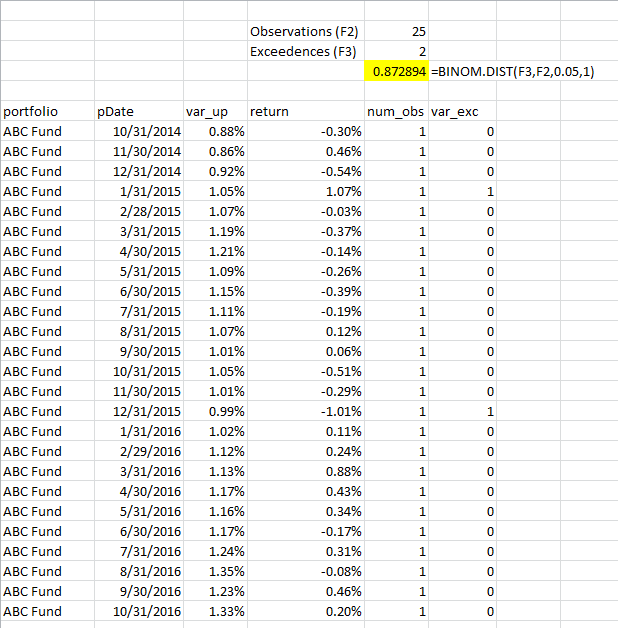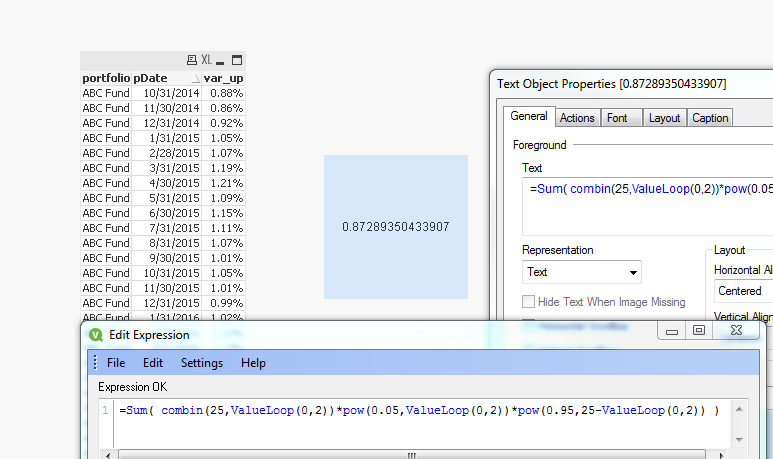# New to Qlik Sense

Discussion board where members can get started with Qlik Sense.

Announcements
Qlik® Product Spotlight: Discover what’s possible. Get more from our products.
See for yourself. Register today.
Not applicable

## Cumulative binomial distribution in equation editor

Hi, I am new to Qlik Sense and was not able to figure out how to calculate cumulative binomial distribution in the equation editor.  I would like to have this value in a table.

Qlik sense does not have discrete binomial distribution function either, but that can be calculated using existing functions as per below:

combin(n,x)*pow(0.05,x)*pow(0.95,n-x)

n and x are integers representing the number of observations and the number of statistical test exceedences at a 95% confidence interval.  The above formula works for discrete outcomes, for example, the probability of having exactly 5 out of 100 results be exceedences (n=100 and x=5 plugged into above), however, what I want is the cumulative probability of 5 or fewer exceedences, which would be the above with (n=100, x=0) + (n=100, x=1) + ... + (n=100, x=5).  It would seem like this could be achieved with a loop, but this does not seem to be available in the editor or an ability to create it in a custom function, something like:

cbd = 0

for i= 0 to x

cbd = cbd + combin(n,i)*pow(0.05,i)*pow(0.95,n-i)

next i

Any help with this would be appreciated1 Solution

Accepted Solutions
Not applicable

## Re: Cumulative binomial distribution in equation editor

Seems like you are calculating your numbers currently in the script using the SQL code, and you want to replace it with QS code.

Try

Input:

pDate,

var_up,

return,

num_obs,

var_exc

FROM

[.\BinomCDFexample.xlsx]

(ooxml, embedded labels, header is 3 lines, table is Sheet1)

WHERE LEN(TRIM(portfolio));

Stats:

TotalExceedence,

NumObservations,

Sum(Binom) as CumBinom

Group by portfolio, TotalExceedence, NumObservations;

TotalExceedence,

NumObservations,

combin(NumObservations,iterno()-1)*pow(0.05,iterno()-1)*pow(0.95,NumObservations-(iterno()-1)) as Binom

While iterno()-1 <= TotalExceedence;

sum(var_exc) as TotalExceedence,

Count(portfolio) as NumObservations

RESIDENT Input

Group by portfolio;

17 Replies
Not applicable

## Re: Cumulative binomial distribution in equation editor

Would you be able to provide few rows of data with the numerical output you expect to see from it?

Not applicable

## Re: Cumulative binomial distribution in equation editor

Please add sample data and sample output

Not applicable

## Re: Cumulative binomial distribution in equation editor

Hi,  thanks for the responses.  Note that I did find a way to get what I want into the report, but it involved some very convoluted SQL involving functions from this post (Cumulative distributive functions for normal and binomial).  I did not handle this on the Qlik side, although I believe it should be possible, so I still would be appreciative if someone could show a way to do it in Qlik (given the data in the table below).  Here is a contrived example, two years of monthly return data, 25 total observations.  There is a measure called VaR which asserts that if the risk model is accurate, the absolute value of the monthly return should exceed VaR in only about 5% of cases. In this example, there are two observations where the realized return exceed the VaR amount.  The cumulative binomial distribution is a hypothesis test saying that the probability of having 2 or fewer exceedences out of 25 observations (given an accurate model) is 87%.  That is the number I am trying to get.  In Excel, it is one formula as per below, in SQL server it is involves coding as per above link.  In Qlik, it is possible to get a discrete probability or the probability of having exactly 2 exceedences:

combin(n,x)*pow(0.05,x)*pow(0.95,n-x)

where n=25 and x=2.  If it would possible to take the aggregation of this formula for x=0, x=1, x=2, then I would get my answer:Hope that more clear and thanks in advance for any help.

Not applicable

## Re: Cumulative binomial distribution in equation editor

Would you be able to attach the Excel file here?

Not applicable

## Re: Cumulative binomial distribution in equation editor

Hi Sunny,  sure, see attached.  I use random numbers for the returns in the example, so column D will change if you recalculate something or hit F9. Note that I am hard coding the 5% threshold, but the number of observations and exceedences will change for different funds / history used, so a solution would have to be flexible there.

Not applicable

## Re: Cumulative binomial distribution in equation editor

I sort off understand the whole thing except these two numbers. What is the logic behind these two numbers?Not applicable

## Re: Cumulative binomial distribution in equation editor

=Sum( combin(25,ValueLoop(0,2))*pow(0.05,ValueLoop(0,2))*pow(0.95,25-ValueLoop(0,2)) )

?

Not applicable

## Re: Cumulative binomial distribution in equation editor

Super!! that did seem to have workedHow exactly is Combin (Combination) function works here?

Not applicable

## Re: Cumulative binomial distribution in equation editor

Community Browser